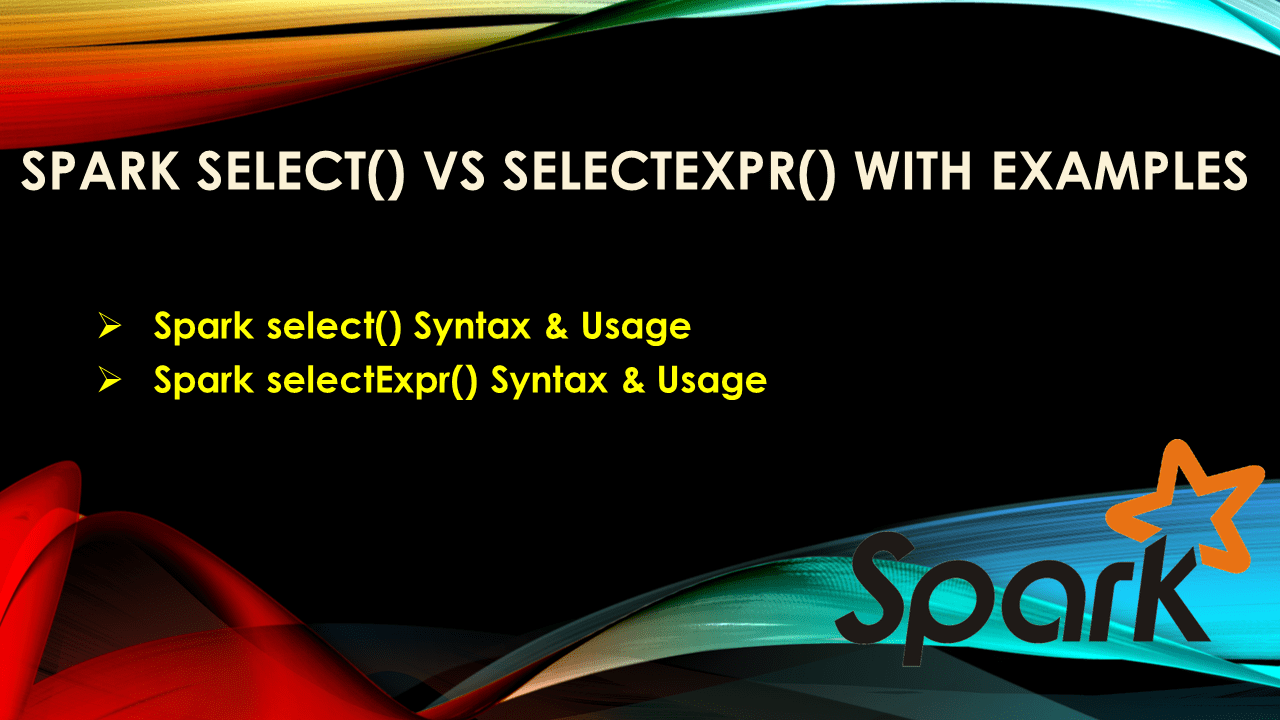# Spark select() vs selectExpr() with Examples

Spark SQL `select()` and `selectExpr()` are used to select the columns from DataFrame and Dataset, In this article, I will explain select() vs selectExpr() differences with examples.

Both these are transformation operations and return a new DataFrame or Dataset based on the usage of UnTyped and Type columns.

• Spark select() Syntax & Usage
• Spark selectExpr() Syntax & Usage

Key points:

## 1. Spark select() Syntax & Usage

Spark `select()` is a transformation function that is used to select the columns from DataFrame and Dataset, It has two different types of syntaxes.

select() that returns DataFrame takes `Column` or String as arguments and used to perform UnTyped transformations.

``````
select(cols : org.apache.spark.sql.Column*) :DataFrame
select(col : scala.Predef.String, cols : scala.Predef.String*) : DataFrame
``````

select() that returns Dataset takes `TypedColumn` as arguments and used for Typed transformations.

``````
select[U1](c1 : TypedColumn[T, U1])
select[U1, U2](c1 : TypedColumn[T, U1], c2 : TypedColumn[T, U2])
``````

#### Example

Below are different ways to select the columns from DataFrame.

``````
val data = Seq(("Java", "20000"), ("Python", "100000"), ("Scala", "3000"))
val df = spark.createDataFrame(data).toDF("language","users_count")

//Example 1
df.select("language","users_count as count")
//Example 2
df.select(df("language"),df("users_count").as("count"))
//Example 3
df.select(col("language"),col("users_count"))
//Example 4
df.select(df.language,df.users_count) //PySpark
``````

It takes either all column names in a String or in a Column type, but you cannot do mix and max. Below returns a compile-time error.

``````
df.select("language",col("users_count"))
``````

## 2. Spark selectExpr() Syntax & Usage

Spark SQL function `selectExpr()` is similar to `select()`, the difference being it takes a set of SQL expressions in a string to execute. This gives an ability to run SQL like expressions without creating a temporary table and views.

`selectExpr()` just has one signature that takes SQL expression in a String and returns a new DataFrame. Note like select() it doesn’t have a signature to take Column type and Dataset return type.

``````
selectExpr(exprs : scala.Predef.String*) : org.apache.spark.sql.DataFrame
``````

#### Example

``````
df.selectExpr("language","users_count as count")
``````

Below is an interesting difference between `select()` vs `selectExpr()`.

``````
Seq(("2019-01-23",1),("2019-06-24",2),("2019-09-20",3))
.toDF("date","increment")
.show()
``````

Alternatively, you can also write the same statement using `expr()` SQL function on select. But the same cannot achieve without expr() function.

``````
Seq(("2019-01-23",1),("2019-06-24",2),("2019-09-20",3))
.toDF("date","increment")
.select(col("date"),col("increment"),